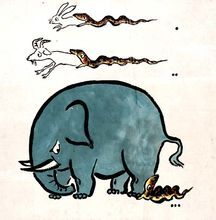# 贪心算法en## 算法思路

1.建立数学模型描述问题

2.把求解的问题分成若干个子问题。

3.对每个子问题求解，得最优解。

4.把子问题的解局部最优解合成原来解问题的一个解。

## 算法例子

### 例子一：分发饼干问题

1.要尽可能多的让孩子得到满足，就是要用最小代价满足孩子的胃口值

2.若是每个饼干都用于满足恰好胃口值的孩子，就能得到最大值

3.每个孩子从小到大的胃口值，被从小到大的饼干满足，这样用的结果就是我们能得到的最大满足孩子个数

func findContentChildren(g []int, s []int) int {	sort.Ints(g)	sort.Ints(s)	var number int //能满足学生总数	var sindex int //已被满足学生坐标	for i := 0; i < len(s); i++ {		if sindex < len(g) && s[i] >= g[sindex] {			number++			sindex++		}	}	return number}

### 例子二：无重叠区间

https://leetcode-cn.com/problems/non-overlapping-intervals/description/?utmsource=LCUS&utmmedium=ipredirect&utmcampaign=transfer2china

1.要无重叠区间，若右闭合一致，区间间隔更长的比区间间隔更短的更容易有重叠，本着贪心思想遇到这种情况直接选择左闭合更大的保留(重复的直接剔除)

2.按照 1 情况处理一遍以后，从小到大的筛选重叠区间就是最优解

func eraseOverlapIntervals(intervals [][]int) int {	if len(intervals)==0{		return 0	}	var number int //需要剔除的区间数目	qmap := make(map[int][]int)//key为区间结尾	rightv:=make([]int,0) //记录右闭合值	for i:=0;i<len(intervals);i++{		if qmap[intervals[i]] == nil{			//这个结尾还没有存在			qmap[intervals[i]] = intervals[i]			rightv = append(rightv,intervals[i])		}else{			//结尾已经存在，如果左闭合更大，说明要替换			if qmap[intervals[i]] < intervals[i]{				qmap[intervals[i]] = intervals[i]			}			number++		}	}	if len(rightv)==1{		return number	}	sort.Ints(rightv)	last:=rightv	for i:=1;i<len(rightv);i++{		if qmap[rightv[i]] < last{			number++		}else{			last = rightv[i]		}	}	return number}

### 例子三：投飞镖刺破气球

1.去除所有同开头，但是右闭合更大的气球，因为他们一定会跟另一个一起爆炸

2.按照开头从小打大，注意每一箭能覆盖的长度范围，左闭合一定小于第一个要射爆气球的右闭合，右闭合取一起射爆气球的最小右闭合。

3.最终结果就是最少可用弓箭数目

func findMinArrowShots(points [][]int) int {	if len(points) == 0 {		return 0	}	qmap := make(map[int][]int) //key为区间结尾	leftv := make([]int, 0)     //记录左闭合值	for i := 0; i < len(points); i++ {		if qmap[points[i]] == nil {			//这个开头还没有存在			qmap[points[i]] = points[i]			leftv = append(leftv, points[i])		} else {			//开头已经存在，如果右闭合更大，说明一定会一起爆，			if qmap[points[i]] > points[i] {				qmap[points[i]] = points[i]			}		}	}	if len(leftv) == 1 {		return 1	}	sort.Ints(leftv)	last := qmap[leftv]	var number int //弓箭数目	number = 1	for i := 1; i < len(leftv); i++ {		if qmap[leftv[i]] <= last {			//可以一起爆，但是要注意边界			if qmap[leftv[i]] < last {				last = qmap[leftv[i]]			}			continue		} else {			//上一轮的结束了			number++			last = qmap[leftv[i]]		}	}	return number}

### 例子四：根据身高重建队列

1.无论怎么解，只要能把单个人放到正确的位置，最后综合的结果就是正确的结论

2.我们按照身高顺序，从高往后排序，先确定高的，将低的按照顺序插入，由于 ki 是身高比他高的人的计数，所以用这个方法可以保证每次都是局部最优，整合是整体最优

func reconstructQueue(people [][]int) [][]int {	if len(people) <= 1 {		return people	}	//0 是身高	//1 是前面比他高的人数	length := len(people)	res := make([][]int, length)	high := make([]int, 0)	pmap := make(map[int][][]int)	for i := 0; i < len(people); i++ {		pmap[people[i]] = append(pmap[people[i]], people[i])		if len(pmap[people[i]]) == 1 {			high = append(high, people[i])		}	}	sort.Ints(high)	for i := len(high) - 1; i >= 0; i-- {    //排序，同高度的要保证k小的优先排序，不然顺序就乱掉了		sort.Slice(pmap[high[i]], func(k, z int) bool {			if pmap[high[i]][k] < pmap[high[i]][z] {				return true			}			return false		})		arrs := pmap[high[i]]		for j := 0; j < len(arrs); j++ {			index := arrs[j]			if res[index] == nil {				res[index] = arrs[j]			} else {				//全部向后一位，没集齐的时候最后一位一定是空				for k := length - 1; k > index; k-- {					res[k] = res[k-1]				}				res[index] = arrs[j]			}		}	}	return res}

### 例子五：经典股票问题

1.从第一天，第二天开始找最优，目标是找到前面最小的股票价钱，再从后面找到与最小股票价钱相差最大的值

2.假设有 7 天，前 3 天股票获取的最大收益如果整个流程没有能超过的，那么前 3 天获得的最优解就是整个流程的最优解

func maxProfit(prices []int) int {	if len(prices) <= 1 {		return 0	}	var max int	//前几日最小的值	min := prices	for i := 1; i < len(prices); i++ {		if prices[i]-min < 0 {			min = prices[i]		} else {			if prices[i]-min > max {				max = prices[i] - min			}		}	}	return max}

### 例子六：非递减数列

1.若是在边界，开头出现 0>1，我们为了递减必然删除 0，计数加 1，结尾出现，处理掉，计数加 1，计数超过 1 则无法达成条件

2.在数组中间，有以下处理方式

1 2 3 5 4 6 7 ==》删除

3 4 2 3 ==》 由于 3>2 所以失败，3 要小于 2，也就是 i-1<i+1，若是 i-1 不小于 i+1，那么可以直接删除 i

2 3 1 5 ==》处理的不再是逆序开始的 i，而是要处理 i+1，不同原因是 i<i+2

func checkPossibility(nums []int) bool {	length := len(nums)	counter := 0	for i := 0; i < length-1; i++ {		if nums[i] > nums[i+1] {			counter++			isOutOfBounds := i != 0 && i+2 < length			if counter > 1 || (isOutOfBounds && nums[i] > nums[i+2] && nums[i-1] > nums[i+1]) {				return false			}		}	}	return true}

### 例子六：最大子序和

1.求最大子序和，那么任何一段的和取最大就是

2.tmp 为中转值，若是 tmp+nums[i]<0 再往下加必然不会是最大，就从 0 开始，又考虑到数组可能为全负数，此种情况就变成了 max 取数组中最大的负数，所以也要考虑

3.若是 tmp+nums[i]>0 就说明加下去还是有可能得出更大的值，拿结果与之前的 max 判断，进行替换。

func maxSubArray(nums []int) int {	max := nums	var tmp int	for i:=0;i<len(nums);i++{		if tmp + nums[i] < 0{			tmp = 0			if nums[i]>max{				max = nums[i]			}		}else{			tmp = tmp + nums[i]			if tmp>max{				max = tmp			}		}	}	return max}

### 例子七：分隔字符串使同种字符出现在一起

1.要尽可能多的分段，那么以最小的字母数为一段，每段区分，所有的集合就是最优解

func partitionLabels(S string) []int {	smap:=make(map[uint8]int)	send:=make(map[uint8]int)	for i:=0;i<len(S);i++{		smap[S[i]]++		send[S[i]] = i	}	var arrs []int	star:=0	for star<len(S){		number:=smap[S[star]]		end:=send[S[star]]		if number+star==end+1{			//数目和字母number一样			arrs = append(arrs,number)			star = end+1		}else{			str:=make(map[uint8]bool)			str[S[star]] = true			for i:=star;i<=end;i++{				if S[star]!=S[i]&&str[S[i]]==false{					//有新字母					str[S[i]] = true					number = number + smap[S[i]]					if send[S[i]] > end{						end = send[S[i]]					}					if end+1==number+star{						arrs = append(arrs,number)						star = end+1						break					}				}			}		}	}	return arrs}

## 参考：## 评论